Low-dose spectral reconstruction with global, local, and nonlocal priors based on subspace decompositionOriginal Article

# Low-dose spectral reconstruction with global, local, and nonlocal priors based on subspace decomposition

Xiaohuan Yu1, Ailong Cai1, Lei Li1, Zhiyong Jiao2, Bin Yan1

1Henan Key Laboratory of Imaging and Intelligent Processing, PLA Strategic Support Force Information Engineering University, Zhengzhou, China; 2Beijing Science and Technology Information Research Center, Beijing, China

Contributions: (I) Conception and design: X Yu; (II) Administrative support: A Cai, L Li, B Yan; (III) Provision of study materials or patients: A Cai, L Li; (IV) Collection and assembly of data: X Yu, A Cai; (V) Data analysis and interpretation: X Yu, A Cai, Z Jiao; (VI) Manuscript writing: All authors; (VII) Final approval of manuscript: All authors.

Correspondence to: Bin Yan. Henan Key Laboratory of Imaging and Intelligent Processing, PLA Strategic Support Force Information Engineering University, No. 62, Science Avenue, High-tech Zone, Zhengzhou 450001, China. Email: ybspace@hotmail.com.

Background: Multienergy computed tomography (MECT) is a promising imaging modality for material decomposition, lesion detection, and other clinical applications. However, there is an urgent need to design efficient and accurate algorithms to solve the inverse problems related to spectral reconstruction and improve image quality, especially under low-dose and incomplete datasets. The key issue for MECT reconstruction is how to efficiently describe the interchannel and intrachannel priors in multichannel images.

Methods: In this model, in order to correlate the similarities of interchannel images and regularize the multichannel images, the global, local, and nonlocal priors are jointly integrated into the low-dose MECT reconstruction model. First, the subspace decomposition method first employs the global low-rankness to map the original MECT images to the low-dimensional eigenimages. Then, nonlocal self-similarity of the eigenimages is cascaded into the optimization model. Additionally, the L0 quasi-norm on gradient images is incorporated into the proposed method to further enhance the local sparsity of intrachannel images. The alternating direction method is applied to solve the optimization model in an iterative scheme.

Results: Simulation, preclinical, and real datasets were applied to validate the effectiveness of the proposed method. From the simulation dataset, the new method was found to reduce the root-mean-square error (RMSE) by 42.31% compared with the latest research fourth-order nonlocal tensor decomposition MECT reconstruction (FONT-SIR) method under 160 projection views. The calculation time of an iteration for the proposed method was 23.07% of the FONT-SIR method. The results of material decomposition in real mouse data further confirmed the accuracy of the proposed method for different materials.

Conclusions: We developed a method in which the global, local, and nonlocal priors are jointly used to develop the reconstruction model for low-dose MECT, where the global low-rankness and nonlocal prior are cascaded by subspace decomposition and block-matching, and the L0 sparsity is applied to express the local prior. The results of the experiments demonstrate that the proposed method based on subspace improves computational efficiency and has advantages in noise suppression and structure preservation over competing algorithms.

Keywords: Multienergy computed tomography (MECT); image reconstruction; global priors; local and nonlocal priors; subspace decomposition

Submitted Jun 21, 2022. Accepted for publication Dec 02, 2022. Published online Jan 05 2023.

doi: 10.21037/qims-22-647

## Introduction

Recently, multienergy computed tomography (MECT) has attracted increasing attention due to its great potential in medical imaging, especially in medicine and surgery, for its capabilities in quantitative material decomposition (1-3). Dual-energy CT (DECT) is one of the most common uses of MECT and can be implemented with dual-energy fast kilovoltage peak (kVp) switching and dual-layer sandwich detectors (4-7). However, it is limited by the use of energy-integrating detectors. The last decade has witnessed the promising development of a new type of MECT equipped with photon-counting detectors (PCDs), which have an energy separation capability in narrow energy bins (8,9). Nevertheless, image qualities are still unsatisfactory due to the low signal-to-noise ratios caused by photon pile-up, charge sharing, fluorescence effect, and photon scattering, which make the material maps suffer from severe noise (10). Therefore, developing efficient and accurate methods to improve image quality is a growing concern in the MECT field.

High-quality reconstruction from noisy and incomplete MECT measurements is a challenging problem. Regularizers encoding the image priors are typically introduced to improve reconstruction stability. For reconstruction in MECT, the popular priors can be categorized as global, local, and nonlocal. Note that the theoretically strict definitions of local and nonlocal priors are unclear. We attempt to classify them according to the ranges of the pixels for computing the regularization function for a target pixel in an image, as detailed in the Appendix 1. With the development of compressed sensing theory (11), many methods based on sparse regularization, such as L0 quasi-norm, total variation (TV) (12), wavelet (13), dictionary learning (14), and other sparse transformations, have been proposed to explore the local property in conventional CT. The sparsity-based regularization can also be used as local priors for each energy bin to reduce image noise in MECT directly. For instance, in 2012, Xu et al. reconstructed the region of interest (ROI) from MECT images by applying a TV penalty (15) to each energy channel. In 2013, Zhao et al. (16) proposed an iterative method based on tight frame (TFIR) to reconstruct higher image quality for sparse-view spectral breast CT. In 2016, Yu et al. (17) extended prior image constraint compressed sensing (PICCS) with TV regularization to spectral CT imaging (spectral PICCS) by introducing a high-quality spectral mean image to improve image quality. In 2018, Niu et al. (18) combined total generalized variation with a prior image to develop an iterative reconstruction for photon-counting CT, and more recently, Wang et al. (19) further used the image-gradient L0 quasi-norm and PICCS algorithm to design a weight adaptive method (L0-ASPICCS) for low-dose MECT in 2020.

Global low-rankness naturally exists in the MECT images due to the strong correlations of the interchannel. To improve the image quality, the local prior and global low-rankness can be modeled in the MECT reconstruction. More precisely, in 2014, Li et al. (20) established a tensor form reconstruction model containing prior rank, intensity, and sparsity (tPRISM) for MECT, which is an extension of PRISM (21). Semerci et al. (22) used the tensor nuclear norm-based iterative reconstruction method to efficiently measure the similarity of MECT images in the spectral domain. In 2015, Rigie and La Rivière (23) further applied the total nuclear variation to reconstruct MECT images with a convex penalty on common edge positions and a shared gradient direction among different channels.

Over the past decade, the idea of nonlocal self-similarity among image patches has been developed as another powerful tool. It has been fully exploited in nonlocal means, block-matching and 3D filtering (BM3D) in image denoising, image recovery, and deblurring (24-26). The research on nonlocal self-similarity also shows promising potential in MECT imaging. In 2015, Kim et al. (27) constructed 3-dimensional patches using self-similarity in the multichannel and carried out sparse-view MECT reconstruction with a low-rank penalty. Moreover, in 2016, as an improved version of dictionary learning, tensor dictionary learning (TDL) was proposed for MECT reconstruction to guarantee effective tissue structure preservation and noise suppression (28). In 2018, Wu et al. (29) combined the L0 quasi-norm with TDL (L0TDL) for low-dose MECT reconstruction, particularly in the sparse view; Niu et al. (30) also use a nonlocal method with low-rank regularization to improve the image quality of MECT. Wu et al. (31) further developed a nonlocal low-rank method based on cube tensor factorization (NLCTF) to obtain better image quality in MECT. More recently, in 2019, Xia et al. (32) proposed a method, called aided by self-similarity in image-spectral tensors (ASSIST), in which each tensor was decomposed into a low-rank component and a sparse component for MECT reconstruction. Hu et al. (33) developed a spectral image similarity-based tensor with an enhanced sparsity reconstruction (SISTER) method by extracting similar blocks that adopted alternating least square-based Candecomp/Parafac (ALS-CP) decomposition (34) to improve the MECT image quality. In 2020, Wu et al. (35) used weight-adaptive TV with spectral tensor factorization in nonlocal prior (WATITF) to improve the image quality of small animal imaging. Zhang et al. (36) combined the CP decomposition with intrinsic tensor sparsity regularization to exploit the nonlocal similarity and expressed spatial sparsity through TV regularization for a PCD-based MECT reconstruction. Chen et al. (37) further proposed a fourth-order nonlocal tensor decomposition model (FONT-SIR) for MECT reconstruction in 2021.

Table 1 is list of some representative methods, including the various priors mentioned above. The methods described tend to adopt different types of priors. The listed methods have achieved relatively better performance than have those of the traditional analytical and typical iterative methods using purely local priors. However, their image qualities for various clinical application are still not adequate, and their use of local and nonlocal priors typically requires high computation complexity .

### Table 1

Summary of a few representative MECT reconstruction methods with different priors

Methods Type of prior
Global Local Nonlocal
TFIR (16)
L0-ASPICCS (19)
tPRISM (20)
Semerci et al. (22)
L0TDL (29)
NLCTF (31)
ASSIST (32)
SISTER (33)
WATITF (35)
Zhang et al. (36)
FONT-SIR (37)
The proposed

MECT, multienergy computed tomography; TFIR, tight frame iterative reconstruction; L0-ASPICCS, L0 norm-based adaptive spectral prior image constrained compressed sensing; tPRISM, tensor prior rank, intensity, and sparsity model; L0TDL, L0 norm-based tensor dictionary learning; NLCTF, nonlocal low-rank cube-based tensor factorization method; ASSIST, aided by self-similarity in image-spectral tensors; SISTER, spectral image similarity-based tensor with enhanced sparsity reconstruction; WATITF, weight-adaptive total variation and image-spectral tensor factorization; FONT-SIR, fourth-order nonlocal tensor decomposition model for spectral CT image reconstruction.

We propose a reconstruction model that exploits the complementary merits of the global, local, and nonlocal priors to improve the image quality with lower computational complexity. High correlations exist in different channels of MECT images, which means that the full MECT images can be expressed or represented by a low-rank subspace (38,39). In this paper, the global and nonlocal regularizations are proposed based on the low-rank subspace decomposition. The most advantageous property of subspace decomposition is that the main features (e.g., detail structures in the image) can be effectively extracted and enhanced, while the global random noise can be suppressed. This method can also improve computational efficiency since the subspace decomposition transfers the global data into a low-rank subspace. In our work, the global low-rankness and nonlocal self-similarity of interchannel images are cascaded through subspace decomposition and nonlocal block-matching. Furthermore, the local spatial sparsity of intrachannel images is also integrated into the model to maintain structure while suppressing noise. The alternating direction method is used to solve the model iteratively.

## Methods

### MECT reconstruction with different priors in parallel

Lowercase letters and boldface lowercase letters (e.g., a and α) are used to denote scalars and vectors, respectively. Matrices and tensors are denoted as capital letters, and calligraphic letters (e.g., A and $X$). $‖⋅‖0$, $‖⋅‖2$ are used to represent the L0 quasi-norm and L2 norm of a vector, respectively. $‖⋅‖F$ is defined as the Frobenius norm of a matrix. Let $X=[x1,x2,...,xs]′∈RS×Np$ represent MECT images of all S channels, where the row $xs, s=1,2,...,S$ of X is the vectorized single-channel CT image with Np pixels. $x∈RS×Np×Np$ denotes the 2-dimensional MECT images of all S channels.

Ignoring the detector response and other effects, the forward projection model is considered the following discretized linear system at S narrow energy windows in MECT:

$Axs+es=bs, s=1,2,...,S$

where $A∈RM×Np$ is the system matrix, $bs∈RM(M=Uy×Nviews)$ stands for the vectorized projections, Uy and Nviews are the number of detector units and projection views, respectively. $es∈RM$ is the noise in the measured projection. For a given system geometry, the task of image reconstruction for MECT is to find xs according to the system geometry and observation bs. However, the reconstruction is ill-posed due to the serious noise in low-dose MECT. It is thus necessary to introduce some priors to build regularization terms to improve the stability of solving the inverse problems.

In order to obtain high-quality reconstruction images, the prior knowledge of the image itself should be introduced to build a regularization model. In this work, we first propose to combine the local and nonlocal regularizations for the low-dose MECT reconstruction problem. The local term is built by the L0 quasi-norm of image gradients and the nonlocal term is established by the block-matching frame-based regularizations, expressed as follows:

$minxs12∑s=1S‖Axs−bs‖22+λ∑s=1S‖∇xs‖0+βR(X)s.t. xs≥0$

where X is the group of all-channel CT images, R (X) represents the regularization term on MECT images based on blocking-matched frames, and $‖∇xs‖0$ denotes the regularization term on single-channel gradient image. λ, β are the nonnegative parameters to balance the data fidelity and regularization term.

In order to decouple the variable X, 2 auxiliary variables, us and Y, are introduced to reformulate the above problem  as follows:

$minxs 12∑s=1S‖Axs−bs‖22+λ∑s=1S‖∇us‖0+βR(Y)s.t. xs=us, X=Y, xs≥0$

Its corresponding augmented Lagrangian function is the following:

,

$12∑s=1S‖Axs−bs‖22+λ∑s=1S‖∇us‖0+βR(Y)+λ12∑s=1S‖xs−us+Λ1λ1‖22+λ22‖X−Y+Λ2λ2‖22$, where $Λ1$ and $Λ2$ are the Lagrange multipliers, and $λ1$ and are the nonnegative parameters.

In this paper, the alternating direction method of multipliers (ADMM) is used to solve the problem . The process is detailed in Algorithm 1, and it is used as a comparison algorithm in the experiments section. It should be noted that, for the subproblem Y, it is actually carried out the nonlocal block-matching denoising process on input data $Yinput=Xl+Λ2l−1λ2$. Due to the effectiveness of suppressing noise, the BM3D algorithm under 3D block-matching frames is selected as a plug-and-play (PnP) module in the reconstruction process (25,40). The algorithm is termed the multienergy BM3D (ME-BM3D) method in this work.

Algorithm 1. ME-BM3D method
Input: parameters $\lambda ,\text{\hspace{0.17em}}{\lambda }_{1},\text{\hspace{0.17em}}{\lambda }_{2},\text{\hspace{0.17em}}\beta ,\text{\hspace{0.17em}}{l}_{\mathrm{max}}$, projection data b.
1. Initialization: ${x}^{0}=0,\text{\hspace{0.17em}}{y}^{0}=0,\text{\hspace{0.17em}}{u}^{0}=0\text{ }{\Lambda }_{1}^{0}={\Lambda }_{2}^{0}=0\text{ }l=0$.
2. While not converged or $l\le {l}_{\mathrm{max}}$
3. ${x}^{l}\in \underset{{x}_{s}}{argmin}\left\{\frac{1}{2}{\sum }_{s=1}^{S}{‖A{x}_{s}-{b}_{s}‖}_{2}^{2}+\frac{{\lambda }_{1}}{2}{\sum }_{s=1}^{S}{‖{x}_{s}-{u}_{s}^{l-1}+\frac{{\Lambda }_{1}^{l-1}}{{\lambda }_{1}}‖}_{2}^{2}+\frac{{\lambda }_{2}}{2}{‖X-{Y}^{l-1}+\frac{{\Lambda }_{2}^{l-1}}{{\lambda }_{2}}‖}_{2}^{2}\right\}$
4. ${u}^{l}\in \underset{{u}_{s}}{argmin}\left\{\lambda {\sum }_{s=1}^{S}{‖\nabla {u}_{s}‖}_{0}+\frac{{\lambda }_{1}}{2}{\sum }_{s=1}^{S}{‖{x}_{s}^{l}-{u}_{s}+\frac{{\Lambda }_{1}^{l-1}}{{\lambda }_{1}}‖}_{2}^{2}\right\}$,
5. ${Y}^{l}\in \underset{Y}{argmin}\left\{\beta R\left(Y\right)+\frac{{\lambda }_{2}}{2}{‖{X}^{l}-Y+\frac{{\Lambda }_{2}^{l-1}}{{\lambda }_{2}}‖}_{2}^{2}\right\}$,
6. ${\Lambda }_{1}^{l}={\Lambda }_{1}^{l-1}+{\lambda }_{1}\left({x}^{l}-{u}^{l}\right),\text{ }{\Lambda }_{2}^{l}={\Lambda }_{2}^{l-1}+{\lambda }_{2}\left({X}^{l}-{Y}^{l}\right)$
7. End while
Output: $\mathcal{X}$

Although the ME-BM3D method takes into account the prior knowledge of the spatial and spectral domain to improve the reconstruction quality, it is solved channelwise in practice, resulting in an increased computational time. Therefore, we further considered the similarity of each channel to design a method based on subspace representation to accelerate MECT reconstruction. Furthermore, it is worth mentioning that the solving methods of $X$ and $U$ subproblems in the ME-BM3D method are consistent with the proposed subspace decomposition-based method introduced in the next subsection.

### Cascaded global and nonlocal priors based on subspace decomposition and block-matching

#### Subspace decomposition

Due to the high correlations between different channels of MECT images, we assume that the images X can be approximated by the k dimensional subspace, where S≥k; that is,

$X=EZ$

where the columns of $E=[e1,e2,...,ek]∈RS×k$ are the basis of subspace Sk. $Z∈Rk×Np$ are the coefficients of X under the basis E. Without loss of generality, we simply assume that E is orthogonal; that is, ET E=Ik, where Ik is an identity matrix of dimension k. Different methods can be followed to infer a subspace matrix E from the MECT images, such as the hyperspectral signal identification by minimum error (Hysime) method (41) or singular value decomposition.

Since E is orthogonal, $Z∈Rk×Np$ can be obtained by projecting MECT images X through subspace E, meaning that Z=ET X. Each row of Z is denoted a vectorized image, and hereafter referred to as eigenimages. According to the literature (38,42), the eigenimages can be denoised with nonlocal patch-based methods due to the nonlocal self-similarity of each eigenimage and the correlation between the eigenimages.

#### Regularization combing with global, local, and nonlocal priors

Images of MECT are highly self-similar. Furthermore, it can be assumed that every single energy image exists in the k-dimensional subspace Sk, where S≥k. Thus, the mathematical model of the MECT reconstruction can be formulated as follows:

$minxs,Z,E12∑s=1S‖Axs−bs‖22+λ∑s=1S‖∇xs‖0+βR(Z)+ρ2‖X−EZ‖F2s.t. ETE=Ik, xs≥0$

The Frobenius norm term represents the difference between subspace decomposition EZ and MECT images X. R (Z) represents the regularization term on eigenimages, and λ, β, ρ are the nonnegative parameters to balance the data fidelity and regularization terms.

The flowchart of the algorithm according to the reconstruction model is presented in Figure 1. Specifically, model  contains the global, local, and nonlocal priors in parallel. Firstly, the subspace decomposition is applied to map the high-dimensional MECT images into a low-dimensional space by using the spectral low-rankness, which corresponds to the fourth term of the objective function in model . Then, the nonlocal similarities are further exploited on each eigenimage by similar block-matching, which is expressed as βR (Z). This step cascades the global low-rankness and the nonlocal priors of interchannel images. Secondly, the denoised eigenimages are aggregated into high-dimensional image space. Finally, the local spatial sparsity is described by L0 quasi-norm regularization, which applies sparsity to a single-channel gradient image.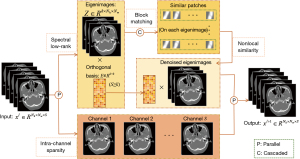Figure 1 The flowchart of the proposed algorithm. The input MECT images are processed through the spectral low-rank, nonlocal block-matching denoising and sparsity regularization of intrachannel to generate the output as the new iteration. MECT, multienergy computed tomography.

#### Details of the solving scheme

For convenience, a third-order tensor $X$ is set to represent all channels of CT images. The $X$ subproblem can be solved through the following optimization:

$minx12∑s=1S‖Axs−bs‖22+λ∑s=1S‖∇xs‖0+ρ2‖X−El−1Zl−1‖F2s.t. xs≥0$

Then, by introducing an auxiliary variable us to replace xs, problem  can be further written into the following constrained form:

$minx,u12∑s=1S‖Axs−bs‖22+λ∑s=1S‖∇us‖0+ρ2‖X−El−1Zl−1‖F2s.t. xs=us, xs≥0$

Based on the augmented Lagrangian function, problem  can be rewritten as follows:

$minx,u,v12∑s=1S‖Axs−bs‖22+λ∑s=1S‖∇us‖0+ρ2‖X−El−1Zl−1‖F2+η2∑s=1S‖xs−us−vs‖22s.t. xs≥0$

where vs is the s-th channel Lagrangian multiplier of $v∈RNp×Np×S$, and η is the nonnegative penalty parameter. Therefore, problem  can be solved via ADMM in the inner loop

$argminx 12∑s=1S‖Axs−bs‖22+ρ2‖X−El−1Zl−1‖F2+η2∑s=1S‖xs−usj−vsj‖22s.t. xs≥0$

$argminu λ∑s=1S‖∇us‖0+η2∑s=1S‖xsj+1−us−vsj‖22$

$vj+1=vj+uj+1−xj+1$

where superscript j, l represent the iteration of the inner and outer loop, respectively. We rearrange X, Z in the second term of Eq.  along the energy channel as xs and zs, and then problem xs can be solved using the separate quadratic surrogate method, which is expressed as follows:

$xsj+1=xsj−csjATA1+ρ+η$

where $csj=AT(Axsj−bs)+ρ(xsj−El−1zsl−1)+η(xsj−usj−vsj)$. The long division stands for pixelwise operation. Meanwhile, the ordered-subset simultaneous algebraic reconstruction technique (OS-SART) (43) is used to accelerate the implementation for xs. Problem  is solved by an approximation algorithm described previously (29). Then, the final output $xsjmax (s=1,2,...,S)$ in the inner loop appears as the l-th iteration Xl of the outer loop. The algorithm of solving $X$ subproblem is summarized in Algorithm 2.

Algorithm 2. ADMM for solving $\mathcal{X}$ subproblem
Input: parameters $\rho ,\text{\hspace{0.17em}}\eta ,\text{\hspace{0.17em}}\lambda ,\text{\hspace{0.17em}}{j}_{max}$, projection data b.
1. Initialization: ${x}^{0}={u}^{0}={v}^{0}=0,\text{ }j=0$.
2. While not converged or $j\le {j}_{max}$
3. Update ${x}^{j+1}$ via 
4. Update ${u}^{j+1}$ via .
5. Update ${v}^{j+1}$ via .
6. End while
Output: ${x}^{l}:={x}^{j+1}$

For E subproblem, the corresponding problem is the following

$El=argminE, ETE=Ik ρ2‖Xl−EZl−1‖F2=L(ξ)S(ξ)T$

where L (ξ), S (ξ) are the left and right singular vectors of the matrix ξ= ρXl (Zl−1)T, respectively (44).

The Z subproblem is transformed into the following optimization scheme:

$Zl=argminZ βR(Z)+ρ2‖Xl−ElZ‖F2=βR(Z)+ρ2‖Z−(El)TXl‖F2$

In this paper, the regularization term of Z acts as a denoiser on the eigenimages rather than on the multienergy CT images. We selected BM3D as the denoiser under the flexible PnP framework. The algorithm is summarized in Algorithm 3.

Algorithm 3. The proposed method for MECT reconstruction
Input: parameters $\rho ,\text{\hspace{0.17em}}\beta ,\text{\hspace{0.17em}}{l}_{\mathrm{max}}$, projection data b.
1. Initialization: ${x}^{0}=0,\text{\hspace{0.17em}}{E}^{0}=0,\text{\hspace{0.17em}}{Z}^{0}=0,\text{ }l=0$.
2. While not converged or $l\le {l}_{\mathrm{max}}$
3. Update ${X}^{l}$ via Algorithm 2.
4. Update ${E}^{l+1}$ via .
5. Update ${Z}^{l+1}$ via .
6. Compute ${X}^{l+1}={E}^{l+1}{Z}^{l+1}$.
7. End while
8. Rearrange vectorized images ${X}^{{l}_{\mathrm{max}}}$ into a third-order tensor ${x}^{{l}_{\mathrm{max}}}$.
Output: $\mathcal{X}$

There are 3 parameters in problem  to balance the data fidelity term, L0 quasi-norm, and the subspace representation term. However, finding the theoretically optimal values is a complex problem. Therefore, we empirically selected them according to experiments. The effect of these parameters on the experiments is presented in the Discussion section in detail. In addition, the $X$ subproblem contains other parameters $η$ to choose from in practice. Its selection was also guided by empirical study. We modified its value according to $τ∑i1i2[ATA]i1i2/∑jNp[us+vs]j$, where $[·]j$ represents the element of matrix or vector, and τ is a nonnegative tuning parameter that was set to 5.7 in the implementation after multiple trials.

## Results

To evaluate the performance of the proposed method, we applied the simulation Moby data, more realistic chest data, and real mouse data to execute the experiments. The multienergy OS-SART (ME-OS-SART) (43), the multienergy BM3D (ME-BM3D; described in Algorithm 1), SISTER (33), WATITF (35), and FONT-SIR (37) methods were considered for comparison. The root-mean-square error (RMSE), structural similarity index (SSIM) (45), and the peak signal-to-noise ratio (PSNR) were employed for quantitative evaluation. In order to verify the effectiveness of the proposed method, material decomposition based on the image-domain was further carried out for the real mouse data.

According to the reconstruction model, there are 3 parameters associated with inter- and intrachannel regularized terms, which are used to balance the fidelity terms. For different datasets (i.e., simulation Moby data, preclinical chest data, and real mouse data), the parameters were not completely consistent because their selections were not only related to the scanning geometry but also depended on the data conditions. In addition, the implementation source code of the comparison methods [SISTER (33), WATITF (35), and FONT-SIR (37)] was given by the relevant authors, and their parameters were thus empirically optimized experimental comparisons and data conditions to ensure the fairness of comparison. All the initial images were set to zero for all methods in experiments. The number of the ordered subsets was set to 10 for all methods that used the accelerated technology. In addition, the iterations were set to 100 for the proposed method. The number of similar patches was 16 with size 8×8, and the search window and stride for patch extraction were 39 and 3, respectively.

### Numerical simulation tests

We first constructed a simulated Moby phantom with the size of 256×256, and each pixel was 0.15 mm × 0.15 mm. To perform multienergy CT reconstruction, the X-ray energy was divided into 8 bins: [16–22), [22–25), [25–28), [28–31), [31–34), [34–37), [37–41) and [41–50) keV. The scanning distances from the source to the object and detector were 132 and 180 mm, respectively. The number of detector units was 512, and the size of each was 0.1 mm. The projection views were set to 80 and 160, and evenly distributed in a 360° range through the circular trajectory. Considering the noise caused by physical effects, such as scattering, stacking, or charge sharing, combined with the law of large numbers and the central limit theorem, random noise was added to the projected data as follows:

$P˜s=Ps+nσmax(Ps)⋅nsns~N(0,1), s=1,2,...,S$

where $Ps, P˜s$ are the clean and noisy single-energy projection, respectively. $ns$ generates a random noise with standard normal distribution, and $nσ$ is the strength parameter which is set to 4/255 here1. To clearly show the structure of Moby data, the reconstructed images of all methods were orientated horizontally and presented as 30×215 pixels.

Figures 2,3 show the reconstructed results of the fourth, sixth, and eighth channels between different methods under 160 and 80 projection views, where columns (A) to (G) represent the images of references, ME-OS-SART, ME-BM3D, WATITF, FONT-SIR, SISTER, and the proposed method, respectively. The extracted ROIs denoted by the yellow dashed square in Figure 2 (A1) and Figure 3 (A1) were magnified below the corresponding reconstructed images. As shown in Figures 2,3, imaging results via ME-OS-SART had the lowest image quality due to its lack of ability to denoise, leading to difficulties in identifying the detailed structures. The ME-BM3D method greatly improved image quality but was limited to preserving image details and fine structures. WATITF, FONT-SIR, and SISTER methods obtained better image quality than the above methods in suppressing noise and preserving structure. However, as pointed out by the orange arrows in the ROIs of Figures 2,3 (G3), some missing details were observed. The proposed method generated high-quality images with subtle detail preservation compared with SISTER and better noise suppression compared with the methods mentioned above. In addition, to verify the robustness of the proposed method against different noises, another set of high-noise experiments is further given. Figure 4 shows the relevant results that the proposed method had a better ability of structure preservation with areas indicated by orange arrows. Compared with other methods, the proposed method maintained the advantages of noise suppression and edge preservation, as indicated by orange arrows, when the noise level was higher.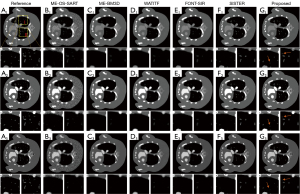Figure 2 Simulated Moby data reconstruction results of different methods under 160 projection views when nσ=4/255. Columns (A) to (G) represent the images of reference, ME-OS-SART, ME-BM3D, WATITF, FONT-SIR, SISTER, and the proposed method. The first 2 rows represent the fourth energy bin and its corresponding ROIs, the middle 2 rows represent the sixth energy bin and its corresponding ROIs, and the bottom 2 rows represent the eighth energy bin and its corresponding ROIs. The display windows of 3 energy bins are [0.01 0.1], [0.01 0.07], [0.01 0.06] mm–1, respectively. The second column indicates the SART results of the low-dose data, which gives a sense of the intensity of the noise. ME-OS-SART, multienergy ordered subset–based simultaneous algebraic reconstruction technique; ME-BM3D, multienergy-based block-matching and 3D filtering; WATITF, weight-adaptive total variation and image-spectral tensor factorization; FONT-SIR, fourth-order nonlocal tensor decomposition model for spectral CT image reconstruction; SISTER, spectral image similarity-based tensor with enhanced sparsity reconstruction; ROI, region of interest; SART, simultaneous algebraic reconstruction technique.Figure 3 Simulated Moby data reconstruction results of different methods under 80 projection views when nσ=4/255. Columns (A) to (G) represent the images of reference, ME-OS-SART, ME-BM3D, WATITF, FONT-SIR, SISTER, and the proposed method. The first 2 rows represent the fourth energy bin and its corresponding ROIs, the middle 2 rows represent the sixth energy bin and its corresponding ROIs, and the bottom 2 rows represent the eighth energy bin and its corresponding ROIs. The display windows of 3 energy bins are [0.01 0.1], [0.01 0.07], [0.01 0.06] mm–1, respectively. ME-OS-SART, multienergy ordered subset-based simultaneous algebraic reconstruction technique; ME-BM3D, multienergy-based block-matching and 3D filtering; WATITF, weight-adaptive total variation and image-spectral tensor factorization; FONT-SIR, fourth-order nonlocal tensor decomposition model for spectral CT image reconstruction; SISTER, spectral image similarity–based tensor with enhanced sparsity reconstruction; ROI, region of interest.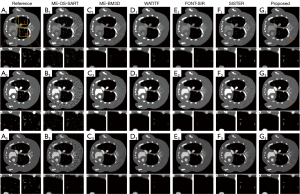Figure 4 Simulated Moby data reconstruction results of different methods under 160 projection views when nσ=20/255. Columns (A) to (G) represent the images of reference, ME-OS-SART, ME-BM3D, WATITF, FONT-SIR, SISTER, and the proposed method. The first 2 rows represent the fourth energy bin and its corresponding ROIs, the middle 2 rows represent the sixth energy bin and its corresponding ROIs, and the bottom 2 rows represent the eighth energy bin and its corresponding ROIs. The display windows of 3 energy bins are [0.01 0.1], [0.01 0.07], [0.01 0.06] mm–1, respectively. ME-OS-SART, multienergy ordered subset-based simultaneous algebraic reconstruction technique; ME-BM3D, multienergy-based block-matching and 3D filtering; WATITF, weight-adaptive total variation and image-spectral tensor factorization; FONT-SIR, fourth-order nonlocal tensor decomposition model for spectral CT image reconstruction; SISTER, spectral image similarity-based tensor with enhanced sparsity reconstruction; ROI, region of interest.

The quantitative assessments of RMSE, PSNR, SSIM, and computational time under 160 views are listed in Table 2. Due to the lack of space, we have only listed the quantitative results of 4 channels between different methods. These results show that the indices of the eighth channel are better than those of the other 3 channels. Compared with other methods, the proposed method achieved the best values in each channel in the RMSE, PSNR, and SSIM indices. Specifically, taking the eighth channel as an example, the proposed method reduced RMSE by 65.91%, 28.48%, 42.31%, 42.31%, and 16.67%, compared with those for the ME-OS-SART, ME-BM3D, WATITF, FONT-SIR, and SISTER methods, respectively. The SSIM and PSNR were as high as 0.9974 and 56.2138 dB for the proposed method, respectively, which were also higher than those of the other methods. Compared with ME-BM3D, WATITF, FONT-SIR, and SISTER, the proposed method had a lower computational cost per iteration, and its calculation time was 90.25%, 42.04%, 23.07%, and 16.42% of the other 4 block-matching-based methods, respectively. The reason the approaches of ME-BM3D, WATITF, FONT-SIR, and SISTER are more time-consuming is that the extraction of similar image patches from all channels of MECT, while the proposed method only clusters these patches in the channels of eigenimages. Both WATITF and our proposed method have 3 priors in the model, but the increase of priors does not imply a corresponding increase in the complexity of our algorithm. The former constructs low-rank cube-based tensors of all channels, and the latter extracts similar patches after dimensionality reduction. By cascading the global and local priors, the global noise can be suppressed and the main structures can be further strengthened, reducing the computational time and avoiding the balance between global and nonlocal priors. The partial line profiles and their enlarged ROIs, drawn from the 120th pixel to the 140th pixel along with the white dashed line in Figure 3 (A1), are further plotted in Figure 5. The proposed method generated more accurate line profiles than did the other methods. As marked by the green arrows, the complex structure of the image is prone to fluctuations of line profiles in the vertical direction, which means that the proposed method is more sensitive to the changes in detail structures.

### Table 2

Quantitative evaluation and computational time of different methods under 160 views

Methods Index Channel 2 Channel 4 Channel 6 Channel 8
ME-OS-SART RMSE 0.0141 0.0081 0.0061 0.0044
PSNR 37.0362 41.7938 44.3267 47.1280
SSIM 0.8556 0.9365 0.9587 0.9750
Time 5.34 seconds (for 1 step iteration)
ME-BM3D RMSE 0.0068 0.0043 0.0035 0.0029
PSNR 43.4139 47.4269 49.1147 50.8172
SSIM 0.9798 0.9882 0.9897 0.9911
Time 7.69 seconds (for 1 step iteration)
WATITF RMSE 0.0082 0.0046 0.0034 0.0026
PSNR 41.7495 46.7385 49.3562 51.7662
SSIM 0.9817 0.9889 0.9911 0.9931
Time 16.51 seconds (for 1 step iteration)
FONT-SIR RMSE 0.0074 0.0043 0.0035 0.0026
PSNR 42.6170 47.3813 49.2002 51.8192
SSIM 0.9815 0.9892 0.9909 0.9929
Time 30.08 seconds (for 1 step iteration)
SISTER RMSE 0.0039 0.0022 0.0019 0.0018
PSNR 48.1268 53.1683 54.3427 55.1248
SSIM 0.9918 0.9961 0.9966 0.9967
Time 42.27 seconds (for 1 step iteration)
Proposed RMSE 0.0036 0.0020 0.0019 0.0015
PSNR 48.8537 54.0383 54.4660 56.2138
SSIM 0.9929 0.9968 0.9969 0.9974
Time 6.94 seconds (for 1 step iteration)

ME-OS-SART, multienergy ordered subset-based simultaneous algebraic reconstruction technique; ME-BM3D, multienergy-based block-matching and 3D filtering; WATITF, weight-adaptive total variation and image-spectral tensor factorization; FONT-SIR, fourth-order nonlocal tensor decomposition model for spectral CT image reconstruction; SISTER, spectral image similarity-based tensor with enhanced sparsity reconstruction; RMSE, root-means-square error; PSNR, peak signal-to-noise ratio; SSIM, structural similarity index.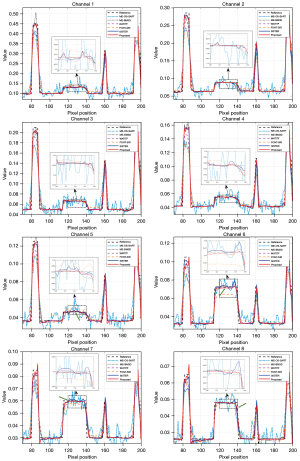Figure 5 Line profiles of 8 channels between different methods under 80 projection views. Rows from top to bottom represent the results of 8 channels. ME-OS-SART, multienergy ordered subset-based simultaneous algebraic reconstruction technique; ME-BM3D, multienergy-based block-matching and 3D filtering; WATITF, weight-adaptive total variation and image-spectral tensor factorization; FONT-SIR, fourthorder nonlocal tensor decomposition model for spectral CT image reconstruction; SISTER, spectral image similarity-based tensor with enhanced sparsity reconstruction.

### Preclinical dataset study

The study was conducted in accordance with the Declaration of Helsinki (as revised in 2013). A public preclinical chest dataset was further chosen to evaluate the performance of the proposed subspace for more realistic medical application scenarios. The data were processed by doctors and contained less noise than did the actual dataset. The tube voltage was 120 kV, and the energy bins were set to [72–80), [78–86), [84–92), [90–98), [96–104), [102–110), [108–116) and [114–120) keV. The size of reconstructed images was 512×512, with each pixel 0.921 mm × 0.921 mm. The number of detector bins was 1024, and the size was 0.69 mm. The distances of the source to the object and the detector were 1,000 and 1,500 mm, respectively. Furthermore, the noise level nσ was set to 0.0382 in this data. To further verify the effectiveness of the proposed method in MECT reconstruction, we conducted experiments on preclinical data with 160 and 80 projection views. Reconstruction results of the SART method, based on 720 noise-free projection views, were taken as the reference of MECT for the subsequent evaluation. Similar to the simulated experiments, we show the 3 energy channels of the reconstructed images at 15×475 pixels horizontally. An ROI [denoted in Figure 6 (A1), labeled I] in the tissue of the chest was magnified to assess the structure preservation across the different algorithms, while another ROI [also denoted in Figure 6 (A1), labeled II] was chosen to make a quantitative evaluation of the mean value of attenuation coefficients and standard deviation (STD), which was calculated as follows:

$STD=1Nroi∑r=1Nroi(xr−x¯)2$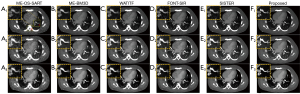Figure 6 The preclinical dataset reconstruction results of different methods under 160 views. Columns (A) to (F) represent the methods of ME-OS-SART, ME-BM3D, WATITF, FONT-SIR, SISTER, and the proposed method, respectively. Rows 1 to 3 represent the energy bins [90–98) keV, [102–110) keV, and [114–120) keV, and the display windows are [0.01 0.11], [0.01 0.07], [0.01 0.05] mm−1, respectively. ME-OS-SART, multienergy-ordered subsets based simultaneous algebraic reconstruction technique; ME-BM3D, multienergy-based block-matching and 3D filtering; WATITF, weight-adaptive total variation and image-spectral tensor factorization; FONT-SIR, fourth-order nonlocal tensor decomposition model for spectral CT image reconstruction; SISTER, spectral image similarity–based tensor with enhanced sparsity reconstruction.

where xr denotes the value of r-th pixel, and x is the precomputed mean value of all Nroi image pixels of the selected ROI.

The reconstructed images and their corresponding enlarged ROIs for 160 projection views obtained by ME-OS-SART, ME-BM3D, WATITF, FONT-SIR, SISTER, and the proposed method are shown in Figure 6, where columns (A) to (F) represent the different approaches, and rows 1 to 3 represent the fourth, the sixth and the eighth channels, respectively. Furthermore, the difference images and the ROIs [denoted in Figure 6 (A1)] are also shown in Figure 7. Figure 6 and Figure 7 (A1)–(A3) show obvious noises in the reconstructions of ME-OS-SART, whereas other methods in Figure 6 and Figure 7 (B1)–(E3) show noise suppression. However, the zoomed-in ROIs show that the ME-BM3D, WATITF, FONT-SIR, and SISTER methods still failed to recover tissue details and edges. Compared with other methods, the proposed method was superior in fine structure preservation and noise suppression. In addition, the results of 80 views, shown in Figure 8 and Figure 9, also demonstrate that the proposed method had the ability to reconstruct high-quality images compared with other methods.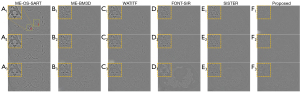Figure 7 The preclinical dataset difference images of different methods under 160 views. Columns (A) to (F) represent the methods of ME-OS-SART, ME-BM3D, WATITF, FONT-SIR, SISTER, and the proposed method, respectively. Rows 1 to 3 represent the energy bins [90–98) keV, [102–110) keV, and [114–120) keV, respectively. The display windows are [–0.15 0.15], [–0.1 0.1], [–0.06 0.06] mm–1, respectively. ME-OS-SART, multienergy-ordered subsets based simultaneous algebraic reconstruction technique; ME-BM3D, multienergy-based block-matching and 3D filtering; WATITF, weight-adaptive total variation and image-spectral tensor factorization; FONT-SIR, fourth-order nonlocal tensor decomposition model for spectral CT image reconstruction; SISTER, spectral image similarity–based tensor with enhanced sparsity reconstruction.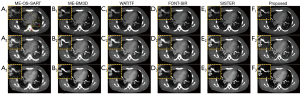Figure 8 The preclinical dataset reconstruction results of different methods under 80 views. Columns (A) to (F) represent the methods of ME-OS-SART, ME-BM3D, WATITF, FONT-SIR, SISTER, and the proposed method, respectively. Rows 1 to 3 represent the energy bins [90–98) keV, [102–110) keV, and [114–120) keV, and the display windows are [0.01 0.11], [0.01 0.07], [0.01 0.05] mm–1, respectively. ME-OS-SART, multienergy-ordered subsets based simultaneous algebraic reconstruction technique; ME-BM3D, multienergy-based block-matching and 3D filtering; WATITF, weight-adaptive total variation and image-spectral tensor factorization; FONT-SIR, fourth-order nonlocal tensor decomposition model for spectral CT image reconstruction; SISTER, spectral image similarity-based tensor with enhanced sparsity reconstruction.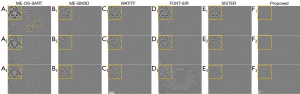Figure 9 The preclinical dataset difference images of different methods under 80 views. Columns (A) to (F) represent the methods of ME-OS-SART, ME-BM3D, WATITF, FONT-SIR, SISTER, and the proposed method, respectively. Rows 1 to 3 represent the energy bins [90–98) keV, [102–110) keV, and [114–120) keV, respectively. The display windows are [–0.15 0.15], [–0.1 0.1], [–0.06 0.06] mm–1, respectively. ME-OS-SART, multienergy-ordered subsets based simultaneous algebraic reconstruction technique; ME-BM3D, multienergy-based block-matching and 3D filtering; WATITF, weight-adaptive total variation and image-spectral tensor factorization; FONT-SIR, fourth-order nonlocal tensor decomposition model for spectral CT image reconstruction; SISTER, spectral image similarity–based tensor with enhanced sparsity reconstruction.

Table 3 lists the quantitative evaluation of different methods under 80 projection views, where the mean value was measured to assess the accuracy of the results, while the STD value evaluated the noise suppression ability of different methods. The STD values of the proposed method, as pointed in superscript asterisk, were close to those for reference images compared with other methods in each energy channel, indicating that the proposed method is superior in suppressing noises. The proposed method had a similar mean value with other methods, indicating the accuracy of reconstruction results.

### Table 3

Means and STDs of different methods for the preclinical dataset under 80 views

Methods Metric Channel 1 Channel 2 Channel 3 Channel 4 Channel 5 Channel 6 Channel 7 Channel 8
Reference Mean value 0.4070 0.1952 0.1319 0.0970 0.0764 0.0610 0.0506 0.0394
STD 0.3895 0.1781 0.1148 0.0766 0.0527 0.0390 0.03 0.0205
ME-OS-SART Mean value 0.4049 0.1955 0.1324 0.0972 0.0769 0.0611 0.0507 0.0395
STD 0.2596 0.1193 0.0764 0.0510 0.0343 0.0258 0.0204 0.0145
ME-BM3D Mean value 0.4032 0.1953 0.1322 0.0972 0.0767 0.0611 0.0506 0.0394
STD 0.3613 0.1664 0.1049 0.0694 0.0466 0.0343 0.0264 0.0177
WATITF Mean value 0.4053 0.1949 0.1318 0.0967 0.0763 0.0609 0.0504 0.0393
STD 0.3161 0.1453 0.0942 0.0630 0.0441 0.0330 0.0258 0.0182
FONT-SIR Mean value 0.3966 0.1938 0.1311 0.0970 0.0776 0.0614 0.0509 0.0406
STD 0.2948 0.1401 0.0908 0.0610 0.0422 0.0310 0.0244 0.0187
SISTER Mean value 0.4029 0.1952 0.1319 0.0971 0.0766 0.0610 0.0506 0.0394
STD 0.3315 0.1532 0.0982 0.0652 0.0446 0.0331 0.0257 0.0175
Proposed Mean value 0.4051 0.1950 0.1316 0.0967 0.0765 0.0611 0.0506 0.0396
STD 0.3757* 0.1730* 0.1118* 0.0746* 0.0511* 0.0384* 0.0301* 0.0219*

*, the indices that close to reference values. ME-OS-SART, multienergy ordered subset-based simultaneous algebraic reconstruction technique; ME-BM3D, multienergy-based block-matching and 3D filtering; WATITF, weight-adaptive total variation and image-spectral tensor factorization; FONT-SIR, fourth-order nonlocal tensor decomposition model for spectral CT image reconstruction; SISTER, spectral image similarity-based tensor with enhanced sparsity reconstruction; STD, standard deviation.

### Real mouse data study

A mouse was scanned through a MARS micro spectral CT system (28,31), which included a micro X-ray source and a flat panel PCD. The study was approved by the Ethics Committee of PLA Strategic Support Force Information Engineering University and was conducted in compliance with the laboratory animal guideline for ethical review of animal welfare. The distances between the source to object and the PCD were 158 and 255 mm, respectively. The length of PCD was 56.32 mm and included 512 pixels, resulting in a field of view with a diameter of 34.69 mm. Gold nanoparticles (GNPs) were injected into the mouse as the contrast agent. Due to the PCD only consisting of 2 energy bins, multiple scans were performed to obtain 13 channels for 371 views with an increasing radiation dose. We extracted the projections for the central slice to reconstruct each channel image with the size of 512×512 in this experiment. We chose 360 and 180 projection views evenly distributed in 371 ranges to verify the proposed method in low-dose spectral CT reconstruction.

Figure 10 shows the reconstruction results of 3 representative energy bins (1st, 7th, 13th) via different methods under 360 views. The first column denotes the images reconstructed by ME-OS-SART with severe noises, but it is difficult to distinguish some soft tissue details. Columns (B) to (E) represent the reconstructions of ME-BM3D, WATITF, FONT-SIR and SISTER, and it seems that a lot noise has been suppressed. However, in the magnified regions, denoted by yellow dashed lines in Figure 10 (A1), the 2 ROIs reconstructed by ME-BM3D, WATITF, FONT-SIR and SISTER indicate a lower ability to preserve bone structures compared with the proposed method. Figure 11 is the reconstructions of the different methods under 180 views and shows similar results.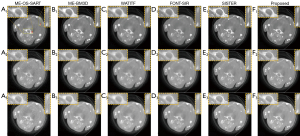Figure 10 The real mouse reconstruction results of different methods under 360 views. Columns (A) to (F) represent the images of ME-OS-SART, ME-BM3D, WATITF, FONT-SIR, SISTER, and the proposed method, respectively. Rows 1 to 3 represent the 1st, 7th, 13th energy bins, respectively. The display windows are [0 0.08], [0 0.07], [0 0.07] mm–1, respectively. ME-OS-SART, multienergy ordered subset-based simultaneous algebraic reconstruction technique; ME-BM3D, multienergy-based block-matching and 3D filtering; WATITF, weight-adaptive total variation and image-spectral tensor factorization; FONT-SIR, fourth-order nonlocal tensor decomposition model for spectral CT image reconstruction; SISTER, spectral image similarity-based tensor with enhanced sparsity reconstruction.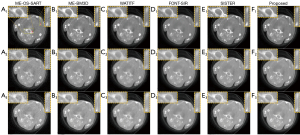Figure 11 The real mouse reconstruction results of different methods under 180 views. Columns (A) to (F) represent the images of ME-OS-SART, ME-BM3D, WATITF, FONT-SIR, SISTER, and the proposed method, respectively. Rows 1 to 3 represent the 1st, 7th, 13th energy bins, respectively. The display windows are [0 0.08], [0 0.07], [0 0.07] mm–1, respectively. ME-OS-SART, multienergy ordered subset-based simultaneous algebraic reconstruction technique; ME-BM3D, multienergy-based block-matching and 3D filtering; WATITF, weight-adaptive total variation and image-spectral tensor factorization; FONT-SIR, fourth-order nonlocal tensor decomposition model for spectral CT image reconstruction; SISTER, spectral image similarity-based tensor with enhanced sparsity reconstruction.

Furthermore, the basis for material decomposition depends on the reconstructed image quality: the better the image quality, the easier the task of postprocessing material decomposition. The first 3 rows in Figure 12 and Figure 13 show the 3 decomposed basis materials: bone, soft tissue, and GNP. The last row includes the colored image, with red, green, and blue representing the 3 materials mentioned above. The decomposition results also show the effectiveness of the proposed method, and the proposed method could provide continuous triangle areas (denoted by red box) of GNP contrast agent with clear image edges. In addition, the bone material decomposed by the proposed method had a more complete and distinct shape, and the probability of misclassifying into GNP was lower than that of the other comparison methods.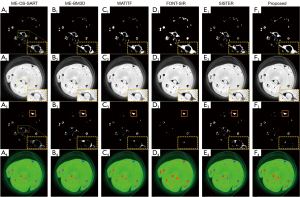Figure 12 The real mouse material decomposition results of different methods under 360 views. Columns (A) to (F) represent the images of ME-OS-SART, ME-BM3D, WATITF, FONT-SIR, SISTER, and the proposed method, respectively. Rows 1 to 3 represent the bone, soft tissue, and GNP, respectively. The fourth row images are the corresponding color rendering, where red, green, and blue represent the above basis materials. The display windows are [0 0.5], [0 1], [0 0.5] cm–1, respectively. ME-OS-SART, multienergy ordered subset–based simultaneous algebraic reconstruction technique; ME-BM3D, multienergy-based block-matching and 3D filtering; WATITF, weight-adaptive total variation and image-spectral tensor factorization; FONT-SIR, fourth-order nonlocal tensor decomposition model for spectral CT image reconstruction; SISTER, spectral image similarity-based tensor with enhanced sparsity reconstruction; GNP, gold nanoparticles.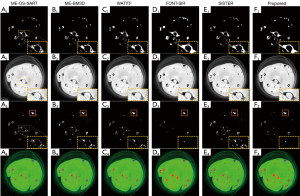Figure 13 The real mouse material decomposition results of different methods under 180 views. Columns (A) to (F) represent the images of ME-OS-SART, ME-BM3D, WATITF, FONT-SIR, SISTER, and the proposed method, respectively. Rows 1 to 3 represent the bone, soft tissue, and GNP, respectively. The fourth row images are the corresponding color rendering, where red, green, and blue represent the above basis materials. The display windows are [0 0.5], [0 1], [0 0.5] cm–1, respectively. ME-OS-SART, multienergy ordered subset-based simultaneous algebraic reconstruction technique; ME-BM3D, multienergy-based block-matching and 3D filtering; WATITF, weight-adaptive total variation and image-spectral tensor factorization; FONT-SIR, fourth-order nonlocal tensor decomposition model for spectral CT image reconstruction; SISTER, spectral image similarity-based tensor with enhanced sparsity reconstruction; GNP, gold nanoparticles.

## Discussion

This section describes some important factors that influenced the implementation of the proposed algorithm in detail, including the dimensions of subspace, the selection of parameters, and the effectiveness of eigenimages denoising.

### Effects of the dimensions of subspace

The influence of the dimensions of subspace k on the MECT reconstruction is discussed through the simulated Moby data under 160 projection views. Since the energy channel of data is 8, the dimensions of the subspace are set to 2, 3, 4, 5, 6, 7, respectively. As shown in Figure 14A, although the quantitative results of different numbers of subspace’s dimensions were similar, the RMSE curve was at the lowest position when k=3. Furthermore, we also explored the computational costs when varying the dimension of subspace, which are shown in Figure 14B. There is no significant consumption of computing time even if the dimension of the subspace is increased. Therefore, we set the dimension of subspace as 3 in our simulation experiments according to the results of RMSE curves and computational costs. Similar to the simulated Moby experiments, the choice of subspace dimensions for preclinical and real data also varied from the corresponding energy windows. Considering the computational time and imaging quality, the number of k could be set as 3, 4 or 5. In practice, we also chose the number 3.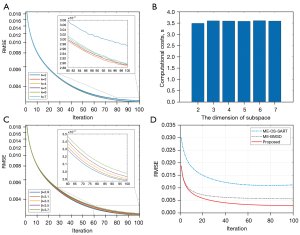Figure 14 Some descriptions of the proposed method. (A) RMSE curves of different dimensions of subspace. (B) Computational costs (unit: seconds) for 1 iteration with different dimensions of subspace. (C) RMSE curves with different values of regularization parameter β. (D) Convergence behaviors of 3 methods (ME-OS-SART, ME-BM3D, and the proposed method). ME-OS-SART, multienergy ordered subset-based simultaneous algebraic reconstruction technique; ME-BM3D, multienergy-based block-matching and 3D filtering; RMSE, root-means-square error.

### Effects of the selection of parameters

There are three parameters in our reconstruction model: λ is used to balance the intrachannel gradient image sparse prior, β is the regularization coefficient interchannel, and ρ is the nonnegative penalty parameter. It may be interesting to study the theoretical analysis of the selection of these parameters. However, we usually make the empirical choices based on the data conditions. In this paper, these parameters are within the range of 10–4 to 104. The RMSE curve changes with different values of β in simulation experiments are shown in Figure 14C. When the ground truth was not available for preclinical and real datasets, we also optimized these parameters according to the data conditions and the evaluations of image quality across several people. The selections of λ, β, and ρ are listed in Table 4 for different datasets.

### Table 4

The parameter values for 3 datasets

Datasets λ β ρ
Simulation 1.8×10–4 2.9 1.1
Preclinical 1.8×10–4 2.9 1.1
Real mouse 1.8×10–6 10 1.1

### Effects of eigenimages Z denoising

β is the regularization parameter of eigenimages Z, which does not directly associate with the original MECT images. In order to verify the effectiveness of the proposed method in eigenimages Z denoising, we chose ME-OS-SART and ME-BM3D as comparison methods, where the ME-BM3D method acted on the reconstructed MECT images by ME-OS-SART. The convergence curves of the 3 methods are shown in Figure 14D. Figure 14D shows that the denoising on eigenimages was more effective than when directly applied to MECT images.

In addition, there is still potential to improve the reconstructed image quality, such as for some detailed structures seem to be lost in the enlarged areas of the proposed method shown in Figure 2 and Figure 3. The appearance of abnormal spikes of channels 6–8 in Figure 5 also indicates that subtle noise was present in some regions, and so how to balance noise suppression and structure preservation also needs further consideration. Furthermore, the regularized terms of the inter- and intrachannel only considers the sparsity of gradient image and the correlations of multichannel images, and the priors can be further explored via integrating a deep denoising network into the MECT reconstruction model, a deep learning has certain advantages in medical image analysis (46-50).

## Conclusions

In this paper, we propose a method to integrate the global, local, and nonlocal priors for low-dose MECT reconstruction in which the global low-rankness and nonlocal priors are cascaded through subspace decomposition and block-matching frames. Subspace representation is used to map original MECT images to a low-dimensional space, and the eigenimages are denoised by BM3D, which greatly reduces the computational complexity. L0 quasi-norm is further applied to exploit the local spatial sparsity in intrachannel images. Then, the model is iteratively solved by the alternating minimization method. Compared with the state-of-the-art methods, the simulation, preclinical, and real data experiments further verified that the proposed method has the ability to improve the performance of denoising and detail preservation.

## Acknowledgments

The authors are grateful to anonymous reviewers for their valuable comments. The authors are also grateful to Dr. Weiwen Wu and Dr. Shaoyu Wang for supplying the real mouse dataset.

Funding: This work was supported by the National Natural Science Foundation of China (No. 62101596) and the National Key Research and Development Program of China (No. 2020YFC1522002). This work was also supported by the China Postdoctoral Science Foundation (No. 2019M663996).

## Footnote

Conflicts of Interest: All authors have completed the ICMJE uniform disclosure form (available at https://qims.amegroups.com/article/view/10.21037/qims-22-647/coif). The authors have no conflicts of interest to declare.

Ethical Statement: The authors are accountable for all aspects of the work in ensuring that questions related to the accuracy or integrity of any part of the work are appropriately investigated and resolved. The study was conducted in accordance with the Declaration of Helsinki (as revised in 2013). The study was approved by the Ethics Committee of PLA Strategic Support Force Information Engineering University, in compliance with the laboratory animal guideline for ethical review of animal welfare.

Open Access Statement: This is an Open Access article distributed in accordance with the Creative Commons Attribution-NonCommercial-NoDerivs 4.0 International License (CC BY-NC-ND 4.0), which permits the non-commercial replication and distribution of the article with the strict proviso that no changes or edits are made and the original work is properly cited (including links to both the formal publication through the relevant DOI and the license). See: https://creativecommons.org/licenses/by-nc-nd/4.0/.

1Due to the complexity of the noise mechanism in the imaging process, we chose the random noise method to simulate the noise in low-dose projections. It was further found that the level of random noise was approximated to 1.4×103 photons in Poisson noise.

1. Bouleti C, Baudry G, Iung B, Arangalage D, Abtan J, Ducrocq G, Steg PG, Vahanian A, Henry-Feugeas MC, Pasi N, Chillon S, Pitocco F, Laissy JP, Ou P. Usefulness of Late Iodine Enhancement on Spectral CT in Acute Myocarditis. JACC Cardiovasc Imaging 2017;10:826-7. [Crossref] [PubMed]
2. Lu X, Lu Z, Yin J, Gao Y, Chen X, Guo Q. Effects of radiation dose levels and spectral iterative reconstruction levels on the accuracy of iodine quantification and virtual monochromatic CT numbers in dual-layer spectral detector CT: an iodine phantom study. Quant Imaging Med Surg 2019;9:188-200. [Crossref] [PubMed]
3. Zhang T, Yu H, Xi Y, Wang S, Liu F. Spectral CT Image-domain Material Decomposition via Sparsity Residual Prior and Dictionary Learning. IEEE T Instrum Meas 2022. doi: 10.1109/TIM.2022.3221120.10.1109/TIM.2022.3221120
4. Graser A, Johnson TR, Chandarana H, Macari M. Dual energy CT: preliminary observations and potential clinical applications in the abdomen. Eur Radiol 2009;19:13-23. [Crossref] [PubMed]
5. Zou Y, Silver MD. Analysis of fast kV-switching in dual energy CT using a pre-reconstruction decomposition technique. Medical Imaging 2008: Physics of Medical Imaging. International Society for Optics and Photonics 2008;6913:691313.
6. Huang X, Gao S, Ma Y, Lu X, Jia Z, Hou Y. The optimal monoenergetic spectral image level of coronary computed tomography (CT) angiography on a dual-layer spectral detector CT with half-dose contrast media. Quant Imaging Med Surg 2020;10:592-603. [Crossref] [PubMed]
7. Wen Q, Yue Y, Shang J, Lu X, Gao L, Hou Y. The application of dual-layer spectral detector computed tomography in solitary pulmonary nodule identification. Quant Imaging Med Surg 2021;11:521-32. [Crossref] [PubMed]
8. Taguchi K, Iwanczyk JS. Vision 20/20: Single photon counting x-ray detectors in medical imaging. Med Phys 2013;40:100901. [Crossref] [PubMed]
9. Shikhaliev PM, Fritz SG. Photon counting spectral CT versus conventional CT: comparative evaluation for breast imaging application. Phys Med Biol 2011;56:1905-30. [Crossref] [PubMed]
10. Leng S, Yu L, Wang J, Fletcher JG, Mistretta CA, McCollough CH. Noise reduction in spectral CT: reducing dose and breaking the trade-off between image noise and energy bin selection. Med Phys 2011;38:4946-57. [Crossref] [PubMed]
11. Candès EJ, Romberg J, Tao T. Robust uncertainty principles: Exact signal reconstruction from highly incomplete frequency information. IEEE T Inform Theory 2006;52:489-509. [Crossref]
12. Sidky EY, Pan X. Image reconstruction in circular cone-beam computed tomography by constrained, total-variation minimization. Phys Med Biol 2008;53:4777-807. [Crossref] [PubMed]
13. Dong B, Li J, Shen Z. X-ray CT image reconstruction via wavelet frame based regularization and Radon domain inpainting. J SCI Comput 2013;54:333-49. [Crossref]
14. Xu Q, Yu H, Mou X, Zhang L, Hsieh J, Wang G. Low-dose X-ray CT reconstruction via dictionary learning. IEEE Trans Med Imaging 2012;31:1682-97. [Crossref] [PubMed]
15. Xu Q, Yu H, Bennett J, He P, Zainon R, Doesburg R, Opie A, Walsh M, Shen H, Butler A, Butler P, Mou X, Wang G. Image reconstruction for hybrid true-color micro-CT. IEEE Trans Biomed Eng 2012;59:1711-9. [Crossref] [PubMed]
16. Zhao B, Gao H, Ding H, Molloi S. Tight-frame based iterative image reconstruction for spectral breast CT. Med Phys 2013;40:031905. [Crossref] [PubMed]
17. Yu Z, Leng S, Li Z, McCollough CH. Spectral prior image constrained compressed sensing (spectral PICCS) for photon-counting computed tomography. Phys Med Biol 2016;61:6707-32. [Crossref] [PubMed]
18. Niu S, Zhang Y, Zhong Y, Liu G, Lu S, Zhang X, Hu S, Wang T, Yu G, Wang J. Iterative reconstruction for photon-counting CT using prior image constrained total generalized variation. Comput Biol Med 2018;103:167-82. [Crossref] [PubMed]
19. Wang S, Wu W, Feng J, Liu F, Yu H. Low-dose spectral CT reconstruction based on image-gradient L(0)-norm and adaptive spectral PICCS. Phys Med Biol 2020;65:245005. [Crossref] [PubMed]
20. Li L, Chen Z, Wang G, Chu J, Gao H. A tensor PRISM algorithm for multi-energy CT reconstruction and comparative studies. J Xray Sci Technol 2014;22:147-63. [Crossref] [PubMed]
21. Gao H, Yu H, Osher S, Wang G. Multi-energy CT based on a prior rank, intensity and sparsity model (PRISM). Inverse Probl 2011; [Crossref] [PubMed]
22. Semerci O, Hao Ning, Kilmer ME, Miller EL. Tensor-based formulation and nuclear norm regularization for multienergy computed tomography. IEEE Trans Image Process 2014;23:1678-93. [Crossref] [PubMed]
23. Rigie DS, La Rivière PJ. Joint reconstruction of multi-channel, spectral CT data via constrained total nuclear variation minimization. Phys Med Biol 2015;60:1741-62. [Crossref] [PubMed]
24. Buades A, Coll B, Morel JM. A non-local algorithm for image denoising. IEEE Computer Society Conference on Computer Vision and Pattern Recognition 2005;2:60-5.
25. Dabov K, Foi A, Katkovnik V, Egiazarian K. Image denoising by sparse 3-D transform-domain collaborative filtering. IEEE Trans Image Process 2007;16:2080-95. [Crossref] [PubMed]
26. Xu J, Zhang L, Zuo W, Zhang D, Feng X. Patch group based nonlocal self-similarity prior learning for image denoising. Proceedings of the IEEE International Conference on Computer Vision 2015:244-52.
27. Kim K, Ye JC, Worstell W, Ouyang J, Rakvongthai Y, El Fakhri G, Li Q. Sparse-view spectral CT reconstruction using spectral patch-based low-rank penalty. IEEE Trans Med Imaging 2015;34:748-60. [Crossref] [PubMed]
28. Zhang Y, Mou X, Wang G, Yu H. Tensor-Based Dictionary Learning for Spectral CT Reconstruction. IEEE Trans Med Imaging 2017;36:142-54. [Crossref] [PubMed]
29. Wu W, Zhang Y, Wang Q, Liu F, Chen P, Yu H. Low-dose spectral CT reconstruction using image gradient ℓ (0)-norm and tensor dictionary. Appl Math Model 2018;63:538-57. [Crossref] [PubMed]
30. Niu S, Yu G, Ma J, Wang J. Nonlocal low-rank and sparse matrix decomposition for spectral CT reconstruction. Inverse Probl 2018;34:024003. [Crossref] [PubMed]
31. Wu W, Liu F, Zhang Y, Wang Q, Yu H. Non-Local Low-Rank Cube-Based Tensor Factorization for Spectral CT Reconstruction. IEEE Trans Med Imaging 2019;38:1079-93. [Crossref] [PubMed]
32. Xia W, Wu W, Niu S, Liu F, Zhou J, Yu H, Zhang Y. Spectral CT reconstruction—ASSIST: Aided by self-similarity in image-spectral tensors. IEEE T Comput Imag 2019;5:420-36. [Crossref]
33. Hu D, Wu W, Xu M, Zhang Y, Liu J, Ge R, Coatrieux G. SISTER: Spectral-image similarity-based tensor with enhanced-sparsity reconstruction for sparse-view multi-energy CT. IEEE T Comput Imag 2019;6:477-90.
34. Kolda TG, Bader BW. Tensor decompositions and applications. SIAM Rev 2009;51:455-500. [Crossref]
35. Wu W, Hu D, An K, Wang S, Luo F. A high-quality photon-counting CT technique based on weight adaptive total-variation and image-spectral tensor factorization for small animals imaging. IEEE T Instrum Meas 2020;70:1-14.
36. Zhang W, Liang N, Wang Z, Cai A, Wang L, Tang C, Zheng Z, Li L, Yan B, Hu G. Multi-energy CT reconstruction using tensor nonlocal similarity and spatial sparsity regularization. Quant Imaging Med Surg 2020;10:1940-60. [Crossref] [PubMed]
37. Chen X, Xia W, Liu Y, Chen H, Zhou J, Zhang Y. Fourth-Order Nonlocal Tensor Decomposition Model For Spectral Computed Tomography. 2021 IEEE 18th International Symposium on Biomedical Imaging (ISBI) 2021:1841-5.
38. Zhuang L, Bioucas-Dias JM. Fast hyperspectral image denoising and inpainting based on low-rank and sparse representations. IEEE J Stars 2018;11:730-42. [Crossref]
39. Lin J, Huang TZ, Zhao XL, Jiang TX, Zhuang L. A tensor subspace representation-based method for hyperspectral image denoising. IEEE T Geo SCI Remote 2020;59:7739-57. [Crossref]
40. Venkatakrishnan SV, Bouman CA, Wohlberg B. Plug-and-play priors for model based reconstruction. 2013 IEEE Global Conference on Signal and Information Processing 2013:945-8.
41. Bioucas-Dias JM, Nascimento JMP. Hyperspectral subspace identification. IEEE T Geo SCI Remote 2008;46:2435-45. [Crossref]
42. Zhuang L, Fu X, Ng MK, Bioucas-Dias JM. Hyperspectral image denoising based on global and nonlocal low-rank factorizations. IEEE T Geo SCI Remote 2021;59:10438-54. [Crossref]
43. Wang G, Jiang M. Ordered-subset simultaneous algebraic reconstruction techniques (OS-SART). J X-ray SCI Technol 2004;12:169-77.
44. Cao C, Yu J, Zhou C, Hu K, Xiao F, Gao X. Hyperspectral image denoising via subspace-based nonlocal low-rank and sparse factorization. IEEE J Stars 2019;12:973-88. [Crossref]
45. Wang Z, Bovik AC, Sheikh HR, Simoncelli EP. Image quality assessment: from error visibility to structural similarity. IEEE Trans Image Process 2004;13:600-12. [Crossref] [PubMed]
46. Wu W, Hu D, Cong W, Shan H, Wang S, Niu C, Yan P, Yu H, Vardhanabhuti V, Wang G. Stabilizing deep tomographic reconstruction: Part A. Hybrid framework and experimental results. Patterns (N Y) 2022;3:100474.
47. Wu W, Hu D, Cong W, Shan H, Wang S, Niu C, Yan P, Yu H, Vardhanabhuti V, Wang G. Stabilizing deep tomographic reconstruction: Part B. Convergence analysis and adversarial attacks. Patterns (N Y) 2022;3:100475.
48. Wu W, Hu D, Niu C, Broeke LV, Butler APH, Cao P, Atlas J, Chernoglazov A, Vardhanabhuti V, Wang G. Deep learning based spectral CT imaging. Neural Netw 2021;144:342-58. [Crossref] [PubMed]
49. Zhang W, Zhou Z, Gao Z, Yang G, Xu L, Wu W, Zhang H. Multiple Adversarial Learning based Angiography Reconstruction for Ultra-low-dose Contrast Medium CT. IEEE J Biomed Health Inform 2022; Epub ahead of print. [Crossref] [PubMed]
50. Wu W, Hu D, Niu C, Yu H, Vardhanabhuti V, Wang G. DRONE: Dual-Domain Residual-based Optimization NEtwork for Sparse-View CT Reconstruction. IEEE Trans Med Imaging 2021;40:3002-14. [Crossref] [PubMed]
Cite this article as: Yu X, Cai A, Li L, Jiao Z, Yan B. Low-dose spectral reconstruction with global, local, and nonlocal priors based on subspace decomposition. Quant Imaging Med Surg 2023;13(2):889-911. doi: 10.21037/qims-22-647